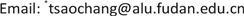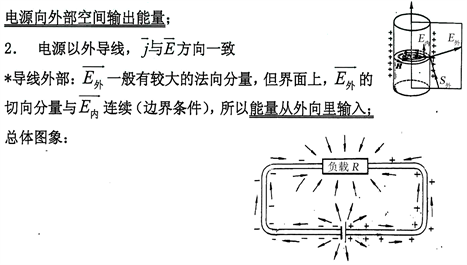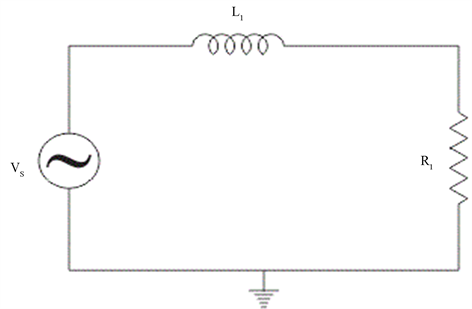﻿ 坡印廷定理的再思考 Rethinking of Poynting’s Theorem

Modern Physics
Vol.08 No.02(2018), Article ID:24190,8 pages
10.12677/MP.2018.82006

Rethinking of Poynting’s Theorem

Tsao Chang1*, Changwei Hu2

1Shanghai Science and Technology Senior University, Shanghai

2Shanghai Senior Scientist Association, ShanghaiReceived: Mar. 2nd, 2018; accepted: Mar. 20th, 2018; published: Mar. 27th, 2018ABSTRACT

In this paper, we study the energy transmission process of direct current and low frequency alternating current. We point out that in addition to the electromagnetic energy flow defined by Poynting vector, there is a “classical” energy transfer process: free electrons driven by the electromotive force of the power supply. The electromotive force of the power supply can be converted into kinetic energy. Outside wires, no current is formed because there are no free electrons. In the case of DC circuits and low-frequency AC circuits, the transfer of electric energy in wires is not related to the displacement current, so it is also independent of the Poynting theorem. The model of lumped element circuit does not calculate the length of the wire. Indeed, it implies an assumption: the speed of low-frequency alternating signals in the wire is infinite. Our experimental results show that under certain conditions, the speed of alternating electrical signals in the wire can be much greater than the speed of light, which is an improvement on the model of lumped parameter circuit.

Keywords:Poynting Theorem, Poynting Vector, DC Circuit, Low Frequency AC Circuit, Longitudinal Electric Field

1上海科技老年大学，上海

2上海市老科协，上海1. 引言Figure 1. Schematic diagram of electromagnetic energy flow in a DC circuit

d 位英国科学家(J.P. Poynting)，他从Maxwell方程组推导出了坡印亭定理   。这个定理类似于经典力学中的能量守恒定理，在数学上类似于连续性方程。

2. 坡印亭定理的推导

$\nabla ×H={j}_{c}+\frac{\partial D}{\partial t}$(1)

$E\cdot \nabla ×H=E\cdot {j}_{c}+E\cdot \frac{\partial D}{\partial t}$ (2)

$\nabla \cdot \left(E×H\right)=-E\cdot \nabla ×H+H\cdot \nabla ×E$ (3)

$H\cdot \nabla ×E-\nabla \cdot \left(E×H\right)=E\cdot {j}_{c}+E\cdot \frac{\partial D}{\partial t}$ (4)

$-\nabla \cdot \left(E×H\right)=E\cdot {j}_{c}+E\cdot \frac{\partial D}{\partial t}+H\cdot \frac{\partial B}{\partial t}$ (5)

$E\cdot \frac{\partial D}{\partial t}=\frac{\partial }{\partial t}\left(\frac{\epsilon {E}^{2}}{2}\right),\text{\hspace{0.17em}}\text{\hspace{0.17em}}H\cdot \frac{\partial H}{\partial t}=\frac{\partial }{\partial t}\left(\frac{\mu {H}^{2}}{2}\right)$ (6)

$-\nabla \cdot \left(E×H\right)=E\cdot {j}_{c}+\frac{\partial }{\partial t}\left(\frac{\epsilon {E}^{2}}{2}+\frac{\mu {H}^{2}}{2}\right)$ (7)

3. 对坡印廷定理的再思考

$\nabla ×H={j}_{c}$ (8)

$E\cdot \nabla ×H=E\cdot {j}_{c}$ (9)

$-\nabla \cdot \left(E×H\right)=\frac{\partial }{\partial t}\left(\frac{\epsilon {E}^{2}}{2}+\frac{\mu {H}^{2}}{2}\right)$ (10)

1) 如果它们是由闭合电路中电源的电动势产生的，那么这个物理过程不涉及到交变磁场产生交变电场，也就不涉及坡印亭定理。

2) 如果是电磁波传输到接收天线，在接收天线的导线内产生了电场强度E和电流密度j，那么这个物理过程需要坡印亭定理。所以，坡印亭定理(7)是有一定适用范围的。

4. RL电路中低频交流电的速度

$U\left(t\right)=I\left(t\right)R+L\text{d}I\left(t\right)/\text{d}t$ (11)Figure 2. A simple RL circuit

$L=2l\left[\mathrm{ln}\left(2l/r\right)-0.75\right]×{10}^{-7}$ (12)

$I\left(t\right)={I}_{o}\mathrm{exp}\left(j\omega t\right)$ (13)

$U\left(t\right)=I\left(t\right)R+j\omega LI\left(t\right)$ (14)

$\omega L\ll R$ 的条件下，式(14)可以改写成为

$U\left(t\right)=I\left(t\right)R\mathrm{exp}\left(j\omega t+j\Phi \right)$ (15)

$\Phi =\omega L/R=\omega \Delta t$ (16)

$\Delta t=L/R$ (17)

${V}_{AC}=l/\Delta t=lR/L$ (18)

5. 结论

1) 如果它们是由闭合电路中电源的电动势产生的，那么这个物理过程不涉及到交变磁场产生交变电场，也就不涉及坡印亭定理。

2) 如果是电磁波传输到接收天线，在接收天线的导线内产生了电场强度E和电流密度j，那么这个物理过程需要坡印亭定理。

Rethinking of Poynting’s Theorem[J]. 现代物理, 2018, 08(02): 42-49. https://doi.org/10.12677/MP.2018.82006

1. 1. 赵凯华, 陈熙谋. 电磁学[M]. 第3版. 北京: 高等教育出版社, 2011: 576-578.

2. 2. Poynting, J.H. (1884) On the Transfer of Energy in the Electromagnetic Field. Philosophical Transactions of the Royal Society of London, 175, 343-361. https://doi.org/10.1098/rstl.1884.0016

3. 3. Poynting, J.H. and Thomson, J.J. (1914) A Text-Book of Physics: Electricity and Mag-netism. Pts. I and II: Static Electricity and Magnetism. C. Griffin, London.

4. 4. 谢处方, 饶克谨. 电磁场和电磁波[M]. 北京: 高等教育出版社, 2005.

5. 5. 胡望雨, 陈秉乾, 舒幼生. 电磁学专题研究[M]. 北京: 高等教育出版社, 2003.

6. 6. Jackson, J.D. (1962) Classical Electrodynamics. John Wiley & Sons Ltd., New York.

7. 7. 蔡圣善, 朱耘. 经典电动力学[M]. 上海: 复旦大学出版社, 1985.

8. 8. Bahl, I. (2003) Lumped Elements for RF and Microwave Circuits. Artech House, London.

9. 9. 张操, 廖康佳, 樊京. 导线中交流电场时间延迟的测定[J]. 现代物理, 2015, 5(2): 29-36.

10. 10. 张操, 廖康佳. 交变电场速度测量的物理原理[J]. 现代物理, 2015, 5(2): 35-39.

11. 11. 张操, 廖康佳, 申红磊, 胡昌伟. 交流电超光速的实验研究[J]. 前沿科学, 2017, 11(1): 67-72.

12. 12. 张操. 关于麦克斯韦方程与经典电路理论的关系[J]. 前沿科学, 2017, 11(3): 24-32.

13. NOTES

*通讯作者。